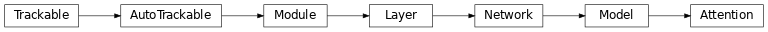# Attention¶

Inheritance Diagramclass ashpy.layers.attention.Attention(filters)[source]

Bases: tensorflow.python.keras.engine.training.Model

Attention Layer from Self-Attention GAN .

First we extract features from the previous layer:

$f(x) = W_f x$
$g(x) = W_g x$
$h(x) = W_h x$

Then we calculate the importance matrix:

$\beta_{j,i} = \frac{\exp(s_{i,j})}{\sum_{i=1}^{N}\exp(s_{ij})}$

$$\beta_{j,i}$$ indicates the extent to which the model attends to the $$i^{th}$$ location when synthethizing the $$j^{th}$$ region.

Then we calculate the output of the attention layer $$(o_1, ..., o_N) \in \mathbb{R}^{C \times N}$$:

$o_j = \sum_{i=1}^{N} \beta_{j,i} h(x_i)$

Finally we combine the (scaled) attention and the input to get the final output of the layer:

$y_i = \gamma o_i + x_i$

where $$\gamma$$ is initialized as 0.

Examples

• Direct Usage:

x = tf.ones((1, 10, 10, 64))

# instantiate attention layer as model
attention = Attention(64)

# evaluate passing x
output = attention(x)

# the output shape is
# the same as the input shape
print(output.shape)

• Inside a Model:

def MyModel():
inputs = tf.keras.layers.Input(shape=[None, None, 64])
attention = Attention(64)
return tf.keras.Model(inputs=inputs, outputs=attention(inputs))

x = tf.ones((1, 10, 10, 64))
model = MyModel()
output = model(x)

print(output.shape)

(1, 10, 10, 64)

  Self-Attention Generative Adversarial Networks https://arxiv.org/abs/1805.08318

Methods

 __init__(filters) Build the Attention Layer. call(inputs[, training]) Perform the computation.

Attributes

 activity_regularizer Optional regularizer function for the output of this layer. dtype dynamic inbound_nodes Deprecated, do NOT use! Only for compatibility with external Keras. input Retrieves the input tensor(s) of a layer. input_mask Retrieves the input mask tensor(s) of a layer. input_shape Retrieves the input shape(s) of a layer. input_spec Gets the network’s input specs. layers losses Losses which are associated with this Layer. metrics Returns the model’s metrics added using compile, add_metric APIs. metrics_names Returns the model’s display labels for all outputs. name Returns the name of this module as passed or determined in the ctor. name_scope Returns a tf.name_scope instance for this class. non_trainable_variables non_trainable_weights outbound_nodes Deprecated, do NOT use! Only for compatibility with external Keras. output Retrieves the output tensor(s) of a layer. output_mask Retrieves the output mask tensor(s) of a layer. output_shape Retrieves the output shape(s) of a layer. run_eagerly Settable attribute indicating whether the model should run eagerly. sample_weights state_updates Returns the updates from all layers that are stateful. stateful submodules Sequence of all sub-modules. trainable trainable_variables Sequence of variables owned by this module and it’s submodules. trainable_weights updates variables Returns the list of all layer variables/weights. weights Returns the list of all layer variables/weights.
__init__(filters)[source]

Build the Attention Layer.

Parameters: filters (int) – Number of filters of the input tensor. It should be preferably a multiple of 8. None
call(inputs, training=False)[source]

Perform the computation.

Parameters: inputs (tf.Tensor) – Inputs for the computation. training (bool) – Controls for training or evaluation mode. Tensor tf.Tensor – Output Tensor.